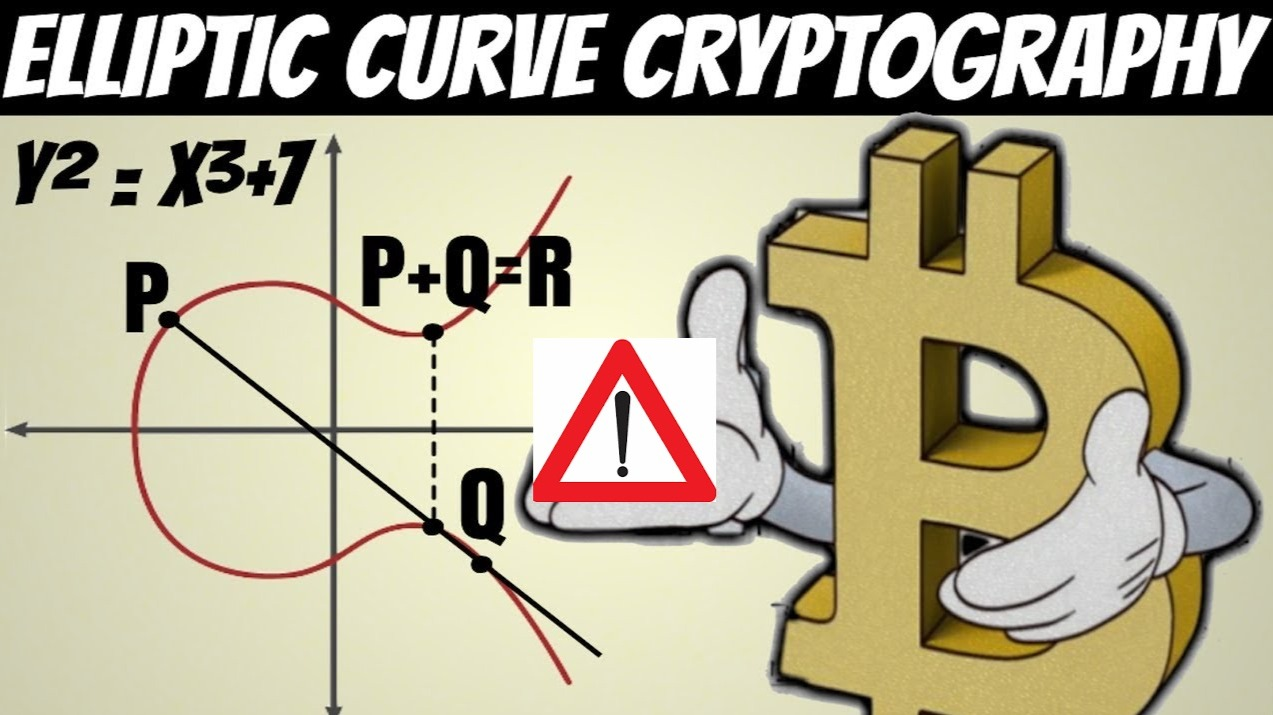# Will Quantum Computer Be Dangerous For Crypto? Elliptic Curves and Shor's Algorithm

Quantum computer, due to their architecture and capabilities, are capable of "breaking" modern cryptography based on the concept of public/private key. It should be stressed though that to date they are still very limited in their functionality (and super expensive for what little they can do). Quantum computers can potentially attack banking systems, cryptocurrencies and especially elliptic curves using an algorithm called "Shor's algorithm". This algorithm can be used to factor prime numbers, which is a key step in many encryption algorithms used to protect data, such as RSA. Elliptic curves are based on cryptocurrencies such as Bitcoin, Ethereum, etc used to generate private/public keys and to encrypt/decrypt transactions. If a powerful enough quantum computer could attack elliptic curves, it could crack private keys and compromise security.

ELLIPTIC CURVES ON BITCOIN
Bitcoin uses secp256k1 which is an elliptic curve. The encryption used by Bitcoin is public and private key (SHA 256). SHA 256 takes input data and produces 256 bit output to hash transaction blocks, it is also used for block integrity and for generating private and public keys.
The key generation process in Bitcoin is as follows:

1) An appropriate elliptic curve (secp256k1) is chosen, defined on a field of prime numbers of 256 bit

2) A random number ("seed") is chosen as the private key, which is kept secret by the owner of the key

3) The corresponding public key is calculated by multiplying the elliptic curve by the seed. This calculation is performed in such a way that only the private key can produce the corresponding public key

The public key is then used to receive payments and sign transactions through the ECDSA algorithm (based on elliptic curves). When a transaction is sent, the sender uses his private key to generate a digital signature proving he is indeed the owner of the keys. The signature is then verified using the sender's public key.
Basically, to simplify the discussion, there is a point on the elliptic curve and a huge scalar number of 256 bit. The two numbers are multiplied, resulting in the other point (R). A modern computer is unable to attack this system, a quantum one, given this point R, is able to calculate the scalar which is the private key of Bitcoin.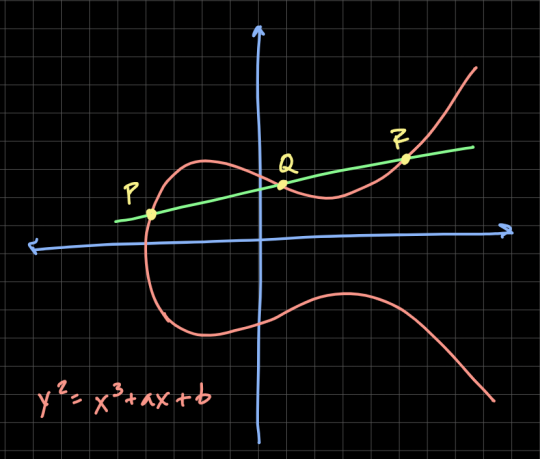More precisely, Shor's algorithm (quantum computers) can be used to quickly factor huge integers into prime factors. Public-key cryptographic algorithms, such as RSA, base their security on the difficulty of factoring large integers into prime factors.
Shor's algorithm uses the quantum property of entanglement to perform an efficient search for the prime factors of an enormously large integer. Instead of using brute force to try every possible factor (by trial and error), as is the case in a classical algorithm, Shor's algorithm uses quantum superposition and interference to search for factors exponentially more efficiently. For example, multiplying two huge prime numbers (P and Q) is done instantly by a calculator or computer. However, if instead of P and Q, the computer has the result R (given by the product of P and Q), it would take billions of years to find the 2 numbers P and Q. Instead a quantum computer thanks to Shor's algorithm, fixes this problem in seconds. Such a quantum computer would destroy not only crypto or the whole internet but also any banking system. Any private key would be found within seconds. Such a system would break  not only the factorization mentioned above (compound number given by 2 prime numbers) but also the elliptic curves. Experimental realization of Shor's quantum factoring algorithm using nuclear magnetic resonance: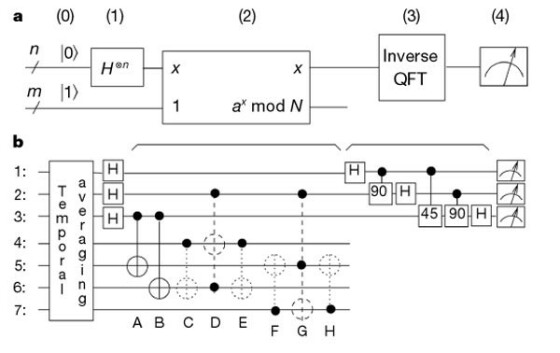The idea would be to build algorithms resistant to the quantum computer. Using elliptic curves, mathematics would have to be completely changed in the event of the advent of quantum computers.

USE OF MULTIPLE ELLIPTICAL CURVES AND MATHEMATICAL LATTICE
There are already quantum-hardy encryption algorithms that can protect cryptocurrencies and elliptic curves, such as Post-Quantum Cryptography (PQC), which uses algorithms based on mathematical problems that are difficult even for a quantum computer to solve. Using multiple elliptic curves can help strengthen the security of Bitcoin transactions. This is possible through the use of a multicurve system, in which multiple elliptic curves are used to generate keys and signatures. By using several elliptic curves, you reduce the risk of a specific curve being compromised by a cryptographic attack, and you can distribute key and signature generation work across multiple curves, improving overall system performance. There would also be greater flexibility based on the specific security and performance needs of the system and greater interoperability of the system with other cryptographic systems based on elliptic curves.
Cryptographic algorithms based on mathematical problems that cannot be solved efficiently by a quantum computer have also been developed. For example, an alternative PQC algorithm to elliptic curve-based cryptography is inherent lattices that exploit the geometry of mathematical lattices for encryption. These cryptographic algorithms are based on the difficulty of finding the shortest vector in a large lattice, known as the "shortest vector problem". This problem is known to be difficult to solve for polynomial-time computers.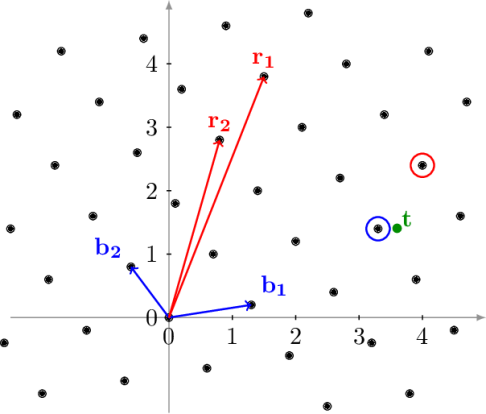Lattices are mathematical geometric structures that can be represented as sets of vectors in an N-dimensional space.
Lattice-based cryptography has been shown to be resistant to quantum computer-based cryptographic attacks, as most of the algorithms known to solve lattice problems require a large amount of computational time and resources to solve.
For example, the NTRU cipher system uses a lattice-based encryption algorithm for key generation and data encryption.
Solving this problem takes exponential time relative to the size of the lattice, making it safe against quantum attacks.

Are you interested in ways to earn crypto bonus? Check it out here: Some Sites To Earn Crypto Bonus (Old & New)☑️Arͬcͨaͣ🅽🅶eͤl̜̝̖̼͔̞̃ͮ͑̐̉͌🅾➏➏➏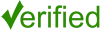I discovered Bitcoin in 2012. I also love NFT. #BTC #ETH #Atom #SNX #Polis #WeAreStarAtlas #MLBSorare⠀⠀⠀⠀⠀⠀Darknet

The topics will be 🅒🅡🅨🅟🅣🅞, of course. I discovered Bitcoin back in 2012.⠀⠀⠀⠀⠀⠀⠀⠀⠀⠀⠀⠀⠀⠀⠀⠀⠀⠀⠀⠀⠀⠀⠀⠀⠀⠀⠀⠀⠀Send a \$0.01 microtip in crypto to the author, and earn yourself as you read!

20% to author / 80% to me.
We pay the tips from our rewards pool.